## When the current in a toroidal solenoid is changing at a rate of 0.0275 a/s, the magnitude of the induced emf is 12.4 mv. when the current e

Question

When the current in a toroidal solenoid is changing at a rate of 0.0275 a/s, the magnitude of the induced emf is 12.4 mv. when the current equals 1.34 a, the average flux through each turn of the solenoid is 0.00285 wb. how many turns does the solenoid have?

in progress 0
6 months 2021-07-27T10:48:49+00:00 1 Answers 5 views 0

41 turns

Explanation: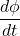= Induced emf = 12.4 mV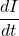= Current changing rate = 0.0275 A/s

L= Inductance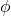= Average flux = 0.00285 wb

N = Number of turns

Change in flux is given by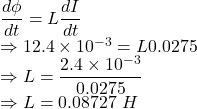Flux through each turn is given by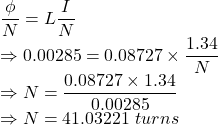The number of turns is 41